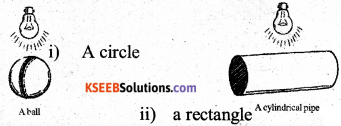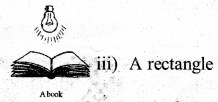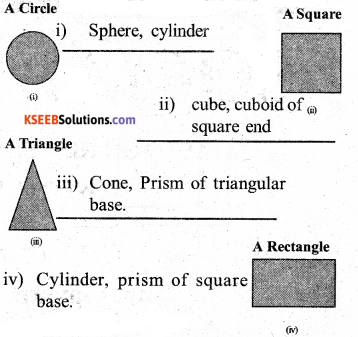# KSEEB Solutions for Class 7 Maths Chapter 15 Visualising Solid Shapes Ex 15.4

Students can Download Chapter 15 Visualising Solid Shapes Ex 15.4, Question and Answers, Notes Pdf, KSEEB Solutions for Class 7 Maths, Karnataka State Board Solutions help you to revise complete Syllabus and score more marks in your examinations.

## Karnataka State Syllabus Class 7 Maths Chapter 15 Visualising Solid Shapes Ex 15.4

Question 1.
A bulb is kept burning just right above the following solids. Name the shape of the shadows obtained in each case. At tempt to give a rough sketch of the shadow. (You may try to experiment first and then answer these questions).Question 2.
Here are the shadows of some 3-D objects, when seen under the lamp of an overhead projector. Identify the solid(s) that match each shadow. (There may be multiple answers for these!)Question 3.
Examine if the following are true statements :
i) The cube can cast a shadow in the shape of a rectangle.
Solution:
True

ii) The cube can cast a shadow in the shape of a hexagon.
Solution:
False.